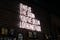# WHAT EXACTLY ARE ARTIFICIAL NEURAL NETWORKS? DETAILED EXPLANATION WITH EXAMPLE.

We know that the whole idea of artificial neural networks is originated from the functioning of the best processor in the world ,that is “the brain”. Through this we are trying to build artificial neurons similar to the biological neurons in our brain and imitate it’s functions. In this article I’m going to explain what actually artificial networks are? What are it’s components? Explaining the layer concept etc.

Moreover to get a better idea I’m going to explain these concepts using the classic example of handwritten digit recognition- the “hello world” of neural networking.

Before getting into artificial ,let’s take…

# ML FUNDAMENTALS:MULTIPLE LINEAR REGRESSION EXPLAINED.

To get a better insight on multiple linear regression ,let me give you a quick walk through Simple linear regression.

SIMPLE LINEAR REGRESSION.

In simple linear regression we only had a single feature and an corresponding result. To be precise there was only one independent and one dependent variable. That is there was only a single input and the relationship between the input and output was one to one. And our hypothesis was represented by

# Machine Learning in Python: Building a Simple Linear Regression Model using SciKit-Learn.

Here comes what you have been looking for!!!!!

An end to end explained, beginner-friendly simple linear regression model using Scikit-learn .I have done my best to explain even minute details to make this article comprehensible for every one.

FIRST AND FORMOST LET ME GIVE YOU A QUICK UNDERSTANDING ABOUT SCIKIT-LEARN :

SciKit-learn is probably the most useful library for machine learning in Python. The sklearn library contains a lot of efficient tools for machine learning and statistical modeling including classification, regression, clustering and dimensionality reduction. This library, which is largely written in Python, is built upon NumPy, SciPy and Matplotlib

# ML FUNDAMENTALS: GRADIENT DESCENT ALGORITHM.

Lets get into our first algorithm in machine learning — GRADIENT DESCENT ALGORITHM.

In the previous articles I have addressed what cost function is and why should it be minimized. In this article I’m going to give an algorithm called gradient descent for that purpose.

If you have no idea about cost function, Checkout my previous articles to get a base in linear regression and cost function.

https://arjun-s.medium.com/simple-linear-regression-ground-level-understanding-e278ebf028d3?source=your_stories_page-------------------------------------

https://arjun-s.medium.com/ml-fundamentals-what-is-cost-function-d396129cc611?source=your_stories_page-------------------------------------

In one sentence gradient descent is an Iterative optimization algorithm that minimizes the cost function or error and finds the best suitable parameters for the hypothesis to perfectly make a fit…

# ML Fundamentals: What Is Cost Function?

It might sound laborious ..but it’s not a big deal. As the name implies it is a function but what is the term “cost” ? Cost simply means error or lost. So lets get into the more deeper side of cost function.

For the sake of understanding better I’m going to explain cost function in terms of simple linear regression. For those who don’t have a basic understanding of simple linear regression check out previous post https://arjun-s.medium.com/simple-linear-regression-ground-level-understanding-e278ebf028d3.

Before starting let me give you a quick recap on hypothesis.

The hypothesis of a simple linear regression model is given below.

# Simple Linear Regression — Ground level understanding.Photo by Austin Chan on Unsplash

Ok ,First of all ,What is Regression?

Regression is a way of predicting one variable from another variable .You know that machine learning is all about predicting the solutions for different problems with the prior training given with help of data sets .Linear regression attempts to model the relationship between two variables by fitting (in the best way possible)a linear equation (= a straight line) to the observed data .The variable which is to be predicted is called dependent variable(Response) and the variable from which we predict the dependent variable is called independent variable (Predictor).

Mathematically ,it is presented as…## Arjun S

Machine learning enthusiasist